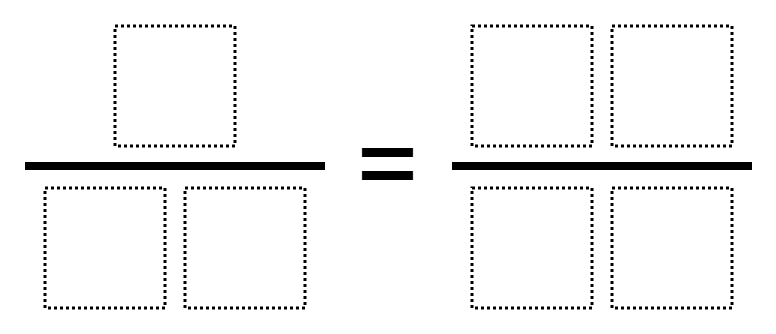# Equivalent Ratios 2

Directions: Using each of the digits 0-6 only once, make two equivalent ratios (also known as a proportion).### Hint

Can multiples help us find equivalent fractions?

Number of Unique Solutions: 6

1: 4/13 = 20/65
2: 4/20 = 13/65
3: 5/10 = 23/46
4: 5/10 = 32/64
5: 5/23 = 10/46
6: 5/32 = 10/64

Source: AnneMarie Untalan

## Solving One-Step Inequalities with Addition

Directions: Using the digits 0 to 9 at most one time each, place a digit …

1.i picked #6 because 5 times 2 =10 and 32 times 2 =64 and together will get 10/64

2.5/23=10/46

•how did you put this in the fraction??

3.I like this website it helps me understand it

4.Yea I also like this website

5.i did 64 times two equals 128

6.4/13 = 20/65

7.3/14 = 6/28

8.5/10=23/46

9.Also, because that was on the answer sheet, I’ll do 10/5=46/23.
Don’t forget…..
…..Improper fractions!

10.4/13=20/65

11.how do you put them in

12.13.14.15.5/10 = 32/64

16.5/23 = 10/46
5/32 = 10/64

17.how do we write them

18.8/24 = 19/57 is another solution. So is 9/21 = 36/84

19.4/8 = 8/16 this is equal bc if u do 4 x 2 it’s –
=‘s 8 then 8 x 2 =16.

20.4/8 = 8/16 this is equal if you do 4 x 2 it’s = 8s then it’s 8×2=16

21.I did 4/8=8/16 because 4*2=8 and 8*2=16 which makes 8/16.

22.I did 4/8=8/16 because 4*2=8 and 8*2=16 which gives you 8/16.